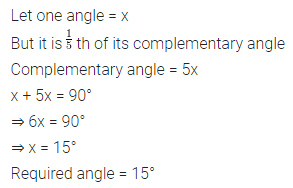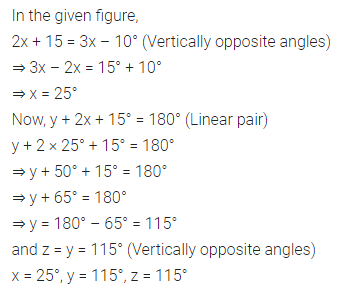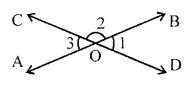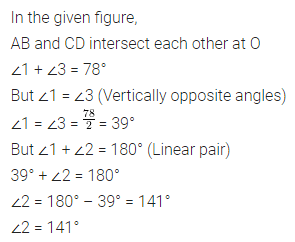# ML Aggarwal Class 7 Solutions for ICSE Maths Chapter 10 Lines and Angles Check Your Progress

## ML Aggarwal Class 7 Solutions for ICSE Maths Chapter 10 Lines and Angles Check Your Progress

Question 1.
Find the supplementary angle of each of the following angles:
(i) $$\frac { 1 }{ 2 }$$ of 90°
(ii) $$\frac { 3 }{ 7 }$$ of 280°
Solution:Question 2.
How many degrees are there in an angle which is one-fifth of its complement?
Solution:Question 3.
If two angles are supplementary and one angle is 5° more than four times the other, find the angles.
Solution:Question 4.
The given diagram shows two intersecting straight lines. Find the values of x, y and z.Solution:Question 5.
In the given diagram, lines AB and CD intersect at O. If ∠1 + ∠3 = 78°, find the size of ∠2.Solution:Question 6.
(a) In the figure (i) given below, l || m. If ∠5 = 65°, find all other angles.
(b) In the figure (ii) given below, l || m. Find the values of x, y and z.
(c) In the figure (iii) given below, l || m and p || q. Find the values of x, y and z.Solution: